# Get the Hour from timestamp in Pandas

• Last Updated : 24 Jul, 2020

Let’s see how to extract the hour from a timestamp in Pandas, with the help of multiple examples.

Example 1 : `pandas.timestamp.now()` takes timezone as input and returns current timestamp object of that timezone.

 `# importing the module``import` `pandas as pd`` ` `# input current timestamp``date ``=` `pd.Timestamp.now()``print``(``"currentTimestamp: "``, date)`` ` `# extract the Hours from the timestamp``frame ``=` `date.hour``print``(``"Hour: "``, frame)`

Output: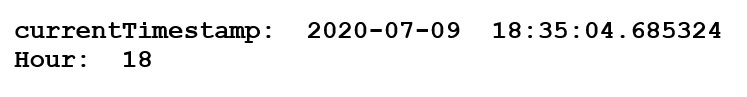Example 2 : `pandas.timestamp()` is used for DateTimeIndex of a specific timezone. It takes year, month, day, time and timezone as input and returns DateTimeIndex of that timezone.

 `# importing the module``import` `pandas as pd   `` ` `# input the timestamp``date ``=` `pd.Timestamp(year ``=` `2020``, month ``=` `7``, day ``=` `21``, ``                    ``hour ``=` `6``, minute ``=` `30``, second ``=` `44``, ``                    ``tz ``=` `'Asia / Kolkata'``)  ``print``(``"Timestamp: "``, date)`` ` `# extract the Hours from the timestamp``print``(``"Hour: "``, date.hour)`

Output :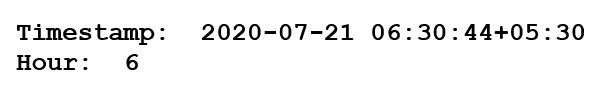Example 3 : Take input as range of timestamps using `pandas.dt_range()` and use `pandas.series()` to convert into an array of timestamps.

 `# importing the module``import` `pandas as pd `` ` `# take input Dates in a range``dates ``=` `pd.Series(pd.date_range(``'2019-8-5 10:23:05'``, periods ``=` `6``, freq ``=``'H'``))`` ` `# convert in a dict container``frame ``=` `pd.DataFrame(``dict``(givenDate ``=` `dates))`` ` `# extract Hours from Timestamp``frame[``'hourOfTimestamp'``] ``=` `frame[``'givenDate'``].dt.hour``print``(frame)`

Output :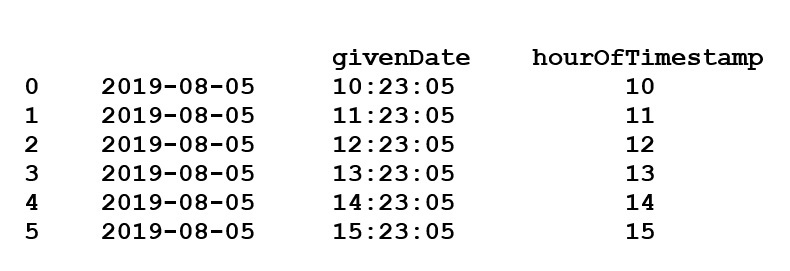Solution 4 : Use `object.hour` attribute to return the hour of the datetime within data of the given Series object.

 `# importing the module``import` `pandas as pd `` ` `# take inputs``dates ``=` `pd.Series([``'2015-01-11 09:20'``, ``'2019-4-8 11:31'``, ``'2018-12-22 10:10'``, ``                   ``'2011-4-2 04:25'``, ``'2017-1-6 03:51'``])  `` ` `# give a Name to the series``seriesName ``=` `[``'T1'``, ``'T2'``, ``'T3'``, ``'T4'``, ``'T5'``]  `` ` `# give index to each timestamp``dates.index ``=` `seriesName`` ` `dates ``=` `pd.to_datetime(dates)`` ` `# extract Hours from Timestamp ``rs ``=` `dates.dt.hour  ``print``(rs)`

Output :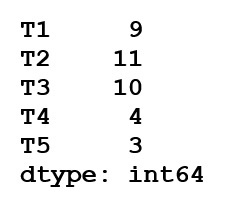Solution 5 : Read timestamp data from a csv file and get Hours from each timestamp.

 `# importing the module``import` `pandas as pd`` ` `# read the date from xyz.csv file``frame ``=` `pd.read_csv(r``'xyz.csv'``)``print``(``"Values in xyz.csv: "``)``print``(frame.head())`` ` `frame[``'dateTime'``] ``=` `frame[``'dateTime'``].astype(``'datetime64[ns]'``)`` ` `# extract Hours from Timestamp  ``print``(``"Hours: "``)``print``(frame.dateTime.dt.hour.head())`

Output :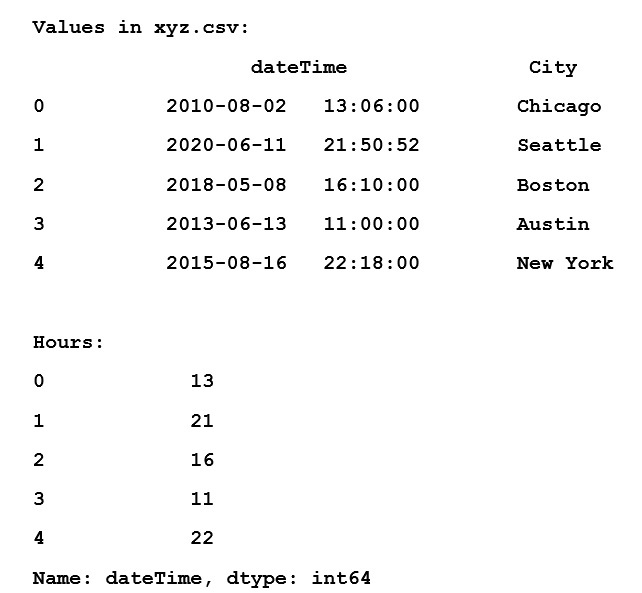My Personal Notes arrow_drop_up